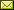## Extensional Models of Untyped Lambda-mu Calculus

 Koji Nakazawa (Graduate School of Informatics, Kyoto University) Shin-ya Katsumata (Research Institute for Mathematical Sciences, Kyoto University)

 This paper proposes new mathematical models of the untyped Lambda-mu calculus. One is called the stream model, which is an extension of the lambda model, in which each term is interpreted as a function from streams to individual data. The other is called the stream combinatory algebra, which is an extension of the combinatory algebra, and it is proved that the extensional equality of the Lambda-mu calculus is equivalent to equality in stream combinatory algebras. In order to define the stream combinatory algebra, we introduce a combinatory calculus SCL, which is an abstraction-free system corresponding to the Lambda-mu calculus. Moreover, it is shown that stream models are algebraically characterized as a particular class of stream combinatory algebras.

In Herman Geuvers and Ugo de'Liguoro: Proceedings Fourth Workshop on Classical Logic and Computation (CL&C 2012), Warwick, England, 8th July 2012, Electronic Proceedings in Theoretical Computer Science 97, pp. 35–47.
Published: 9th October 2012.

 ArXived at: https://dx.doi.org/10.4204/EPTCS.97.3 bibtex PDF
References in reconstructed bibtex, XML and HTML format (approximated).
 Comments and questions to:eptcs@eptcs.org For website issues:webmaster@eptcs.org